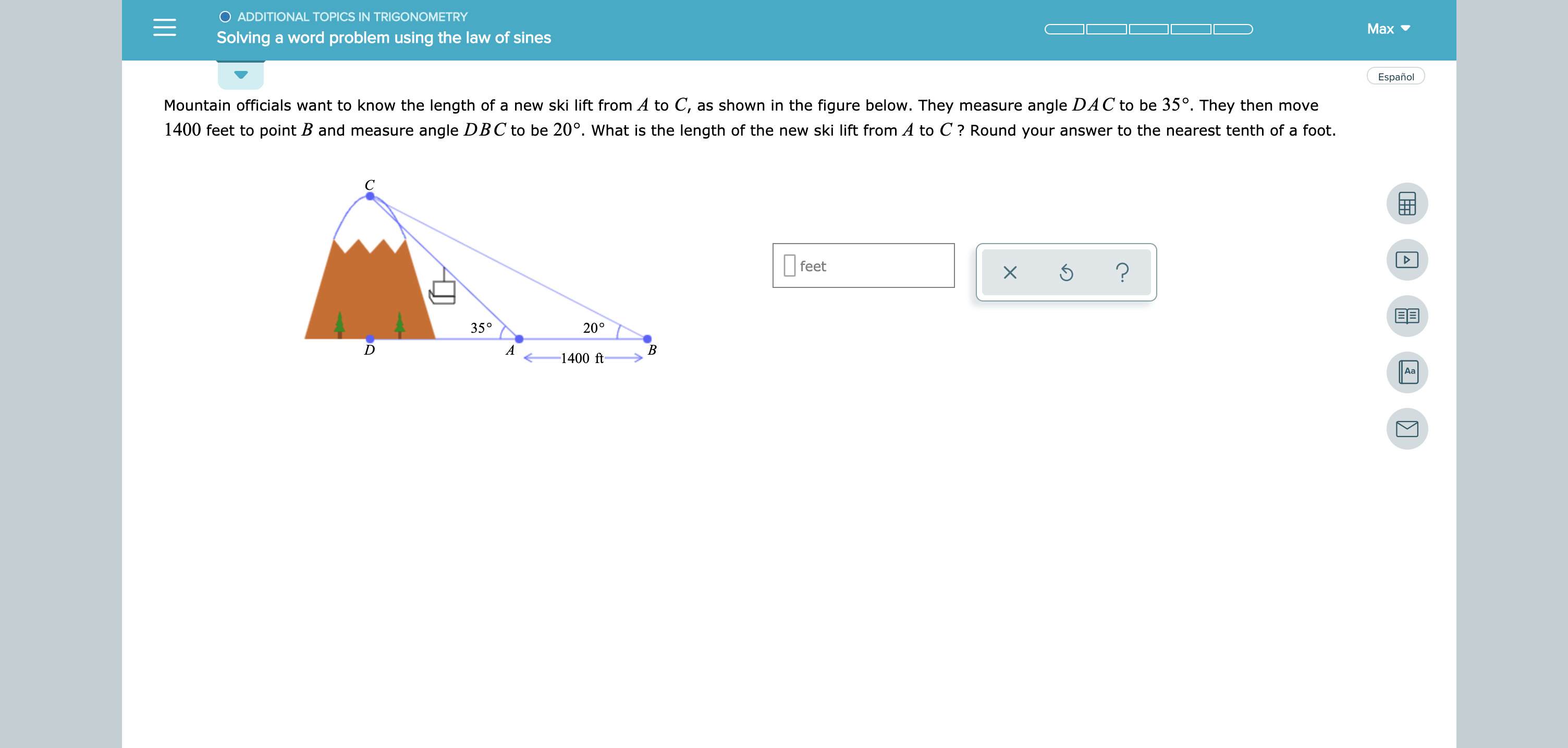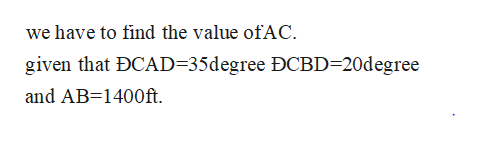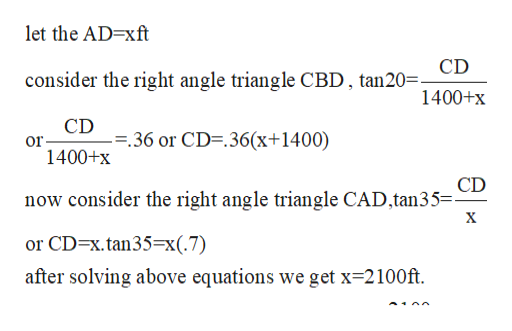ADDITIONAL TOPICS IN TRIGONOMETRYМахSolving a word problem using the law of sinesEspañolMountain officials want to know the length of a new ski lift from A to C,as shown in the figure below. They measure angle DAC to be 35°. They then move1400 feet to point B and measure angle DBC to be 20°. What is the length of the new ski lift from A to C? Round your answer to the nearest tenth of a footfeet?35°20°AВ1400 ftAa

Question

see attachmenthelp_outlineImage TranscriptioncloseADDITIONAL TOPICS IN TRIGONOMETRY Мах Solving a word problem using the law of sines Español Mountain officials want to know the length of a new ski lift from A to C, as shown in the figure below. They measure angle DAC to be 35°. They then move 1400 feet to point B and measure angle DBC to be 20°. What is the length of the new ski lift from A to C? Round your answer to the nearest tenth of a foot feet ? 35° 20° A В 1400 ft Aa fullscreen
Step 1

the value of AC=?help_outlineImage Transcriptionclosewe have to find the value ofAC. given that DCAD=35degree ĐCBD-20degree and AB 1400ft. fullscreen
Step 2

it is the base what we did find. fo...help_outlineImage Transcriptioncloselet the AD-xft consider the right angle triangle CBD, tan20= 1400+x CD --36 or CD-.36(x+1400) or 1400+x CD now consider the right angle triangle CAD,tan35=- X or CD-x.tan35=x(.7) after solving above equations we get x 2100ft fullscreen

Want to see the full answer?

See Solution

Want to see this answer and more?

Our solutions are written by experts, many with advanced degrees, and available 24/7

See Solution
Tagged in

Inverse Trigonometric Ratios